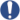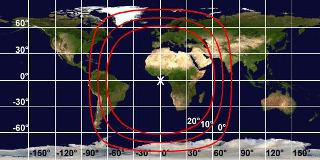# Geostationary orbit

## Where is the geostationary orbit?Automatic translation Category: probes and satellites
Updated June 22, 2014

The geostationary orbit is an orbit in the equatorial plane of the Earth at 35,796 km altitude. Moreover, its orbital eccentricity is zero, which means that it is perfectly circular. This particular case geosynchronous orbit allows an artificial satellite « remain motionless » on the vertical equator at a fixed position relative to any point on the surface of the planet.
Artificial satellite rotates in the same direction as the Earth on its axis, i.e. from west to east (relative to celestial reference system). Then the satellite has an orbital period exactly equal to the period of rotation of the Earth itself, 23 hours 56 minutes and 4.084 seconds, this period is called the sidereal day.
What is the use of the geostationary orbit?
The geostationary orbit is used to position satellites that absolutely must cover a fixed region or to be pointed to by fixed antennas on the ground. The main satellites orbit at 36,000 km altitude are telecommunications satellites, Television Broadcasting, observation and meteorological satellites.
In June 2014 there were 254 satellites in geostationary orbit.

The position of satellites "geostationary" is managed by the International Telecommunication Union. This orbit is relatively encumbered, satellites at the end of life must leave their room for new satellites. Above of the geostationary orbit is a belt 230 km called « disposal orbit » or « dustbin orbit » is the cemetery of satellites at end of life. When a geostationary satellite is no longer active it is sent to this orbit. Geostationary satellites revolve on themselves at a speed of 100 revolutions per minute.

nota: The geostationary orbit is a circular orbit that allows a satellite to make a revolution around the planet while the planet makes one rotation around itself. As its inclination to the equatorial plane of the Earth is equal to 0, the satellite appears « immobile », suspended in the sky always in the same position above the equator. Geostationary satellites are used for continuous observation of a specific area of ​​the globe.## How to calculate the geostationary orbit?

The geostationary orbit is calculated using the law of universal gravitation, which boils down to:
h=√3(G*M*T2/4π2)-R

h = height of the artificial satellite in km
G = constant of universal gravitation (6.67*10-11)
M = mass of the planet kg
T = period of rotation of the planet in seconds
R = radius of the planet in km
The geostationary orbit (synchronous orbit of the Earth) is at an altitude of 35,796 km (≈ 36,000 km) and has a semi-major axis of 42,167 km.

nota: Excel formula used to calculate the altitude of the satellite in synchronous orbit of the planet:
=((((G*M*T^2)/(4*PI()^2))^(1/3))-R*1000)/1000
Excel formula used to calculate the semi-major axis of the synchronous orbit of the planet:
=((((G*M*T^2)/(4*PI()^2))^(1/3)))/1000

### Image: many active satellites are in geostationary orbit at ≈ 36 000 km altitude on the image 254 satellites (June 2014) draw a perfect circle around the Earth. These are mainly telecommunications satellites, television broadcasting, observation and meteorological satellites. Credit astronoo.com.1997 © Astronoo.com − Astronomy, Astrophysics, Evolution and Earth science.What is an apsis?Rosetta and Philae...With or without horizon,
where is the space station?